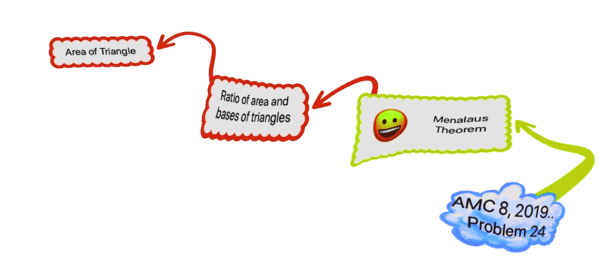Categories

# Menalaus Theorem in AMC 8 2019

Learn how to use Menalaus’s Theorem to solve geometry problem from AMC 8 2019. We also provide Knowledge Graph and a video discussion.

# What are we learning ?

Competency in Focus: Menalaus’s Theorem This problem from American Mathematics contest (AMC 8, 2019) will help us to learn more about Menalaus’s Theorem.

# First look at the knowledge graph.# Next understand the problem

In triangle 𝐴𝐵𝐶, point 𝐷 divides side AC so that 𝐴𝐷 ∶ 𝐷𝐶 = 1 ∶ 2. Let 𝐸 be the midpoint of BD and 𝐹 be the point of intersection of line BC and line AE. Given that the area of ∆𝐴𝐵𝐶 is 360, what is the area of ∆𝐸𝐵𝐹?
##### Source of the problem
American Mathematical Contest 2019, AMC 8 Problem 25
##### Key Competency
Menalaus’s Theorem:   Given a triangle ABC, and a transversal line that crosses BC, AC, and AB at points D, E, and F respectively, with D, E, and F distinct from A, B, and C, then

$$\displaystyle {\frac {AF}{FB}\times \frac {BD}{DC}\times \frac {CE}{EA}=-1.}$$

7/10

##### Suggested Book
Challenges and Thrills in Pre College Mathematics Excursion Of Mathematics

# Connected Program at Cheenta

#### Amc 8 Master class

Cheenta AMC Training Camp consists of live group and one on one classes, 24/7 doubt clearing and continuous problem solving streams.

# Similar Problems## By Dr. Ashani Dasgupta

Ph.D. in Mathematics, University of Wisconsin, Milwaukee, United States.

Research Interest: Geometric Group Theory, Relatively Hyperbolic Groups.

Founder, Cheenta

This site uses Akismet to reduce spam. Learn how your comment data is processed.